# F2O or OF2 lewis dot structure, molecular shape, electron geometry, polar or non-polar, bond angle

Home  > Chemistry Article > OF2 lewis structure and its molecular geometry/shape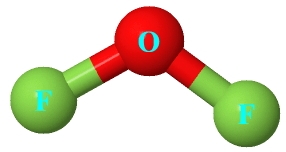Oxygen difluoride is a colorless and very toxic gas with the chemical formula OF2. It is considered very dangerous due to its oxidizing properties.

In this article, we will discuss the Oxygen difluoride (OF2) lewis dot structure or F2O lewis structure, its molecular and electron geometry, bond angle, hybridization, is OF2 polar or non-polar, etc.

OF2 can explode if it contacts water. It is used as an oxidizer for propellants. It has many names like- Difluorine monoxide, Oxydifluoride, Oxygen difluoride, etc.

Some important properties of OF2

1. It is highly toxic by inhalation.
2. It has a molar mass of 53.99 g/mol.
3. It has smelled peculiar.
4. The boiling point of OF2 is −144.75 °C and the melting point is −223.8 °C.
5. It is corrosive to the skin and eyes.

OF2 can be obtained by reacting fluorine with sodium hydroxide in a dilute aqueous solution.

⇒ 2F2 + 2 NaOH → OF2 + 2 NaF + H2O

 Name of Molecule Oxygen difluoride Chemical formula OF2 Molecular geometry of OF2 Bent Electron geometry of OF2 Tetrahedral Hybridization Sp³ Bond angle 103º Total Valence electron for OF2 20 The formal charge of OF2 0
Page Contents

## How to draw Oxygen difluoride (OF2) lewis structure?

OF2 lewis structure is made up of one oxygen atom and two fluorine atoms, the oxygen is in the central position and fluorine atoms on either side of it. The lewis structure of OF2 contains 8 lone pairs(2 lone pairs on oxygen atom and 3 lone pairs on each fluorine atom).

The lewis structure of OF2 is very easy to draw and the procedure for drawing is also simple. Let’s see how to do it.

## Steps to follow for drawing the OF2/F2O lewis structure

1. Count total valence electron present in OF2

In the first step, you need to find how many valence electrons are present in the OF2 molecule. As oxygen belongs to the 16th group in the periodic table and fluorine is in the 17th group.

⇒ Oxygen valence electrons = 6

⇒ Fluorine valence electrons = 7

∴ Total valence electrons available for drawing the OF2 lewis structure = 6 + 7(2) = 20 valence electrons          [∴ OF2 molecule contains one oxygen and two fluorine]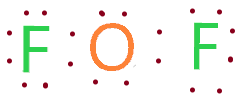2. Find the least electronegative atom in OF2

After finding total valence electrons, we need to find the least electronegative for placing them at the center of the F2O lewis structure.

As electronegativity increase from left to right in the periodic table. So, oxygen is at the left and fluorine at the right in the periodic table. Hence, oxygen is less electronegative than a fluorine atoms.

Place Oxygen at the center in the lewis diagram and fluorine spaced evenly around it.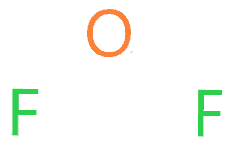3. Connect oxygen and fluorine with a single bond

Now in this step, we will start to draw the Lewis structure of F2O by simply connect a fluorine atom with a central atom with a single bond.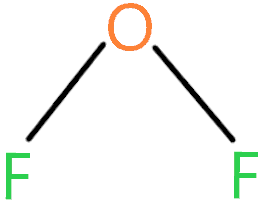∴ (20 – 4) = 16 valence electrons

Now we are left with 16 more valence electrons.

4. Place remaining valence electrons starting from the outer atom first

As we have a total of 16 valence electrons more and we need to complete the octet rule of atoms in this step. Start with the outer atom first.

Fluorine needs 8 electrons to complete its octet rule but it already shares 2 electrons with a single bond. So, it only needs 6 more electrons to fulfill its octet rule.

Place 6 electrons around each fluorine atom in the 3rd step structure.After placing 6 electrons around each fluorine atom we left with 4 valence electrons. We need to use these 4 valence electrons to fulfill the octet for the central atom.

Let’s see in the next step.

5. Complete central atom octet and make covalent bond if necessary

Oxygen needs 8 electrons to complete its octet rule but it already shares 4 electrons with the help of 2 single bonds connected with fluorine atoms.

And we have 4 valence electrons more. So, place these electrons around oxygen and fulfill its octet.### OF2 Lewis structure

So, we used all 20 valence electrons, and all atoms in the OF2 lewis structure are also happy because they completed their octet rule.

No need to make any covalent bond as we got our best lewis dot structure for OF2 (Oxygen difluoride).

## What are the electron and molecular geometry of OF2?

The molecular geometry of OF2 is bent and its electron geometry is tetrahedral because the presence of two lone pairs on the central atom creates repulsion with bonded pairs of electrons, as a result, all outer atoms(fluorine) pushes down in order to minimize the repulsion according to the VSEPR theory, and that makes its shape look bent.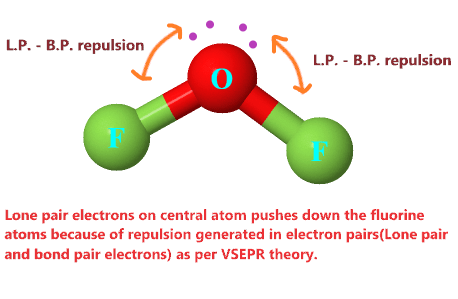Also, the presence of three lone on each fluorine atom repels one another that makes its bond angle somewhat wider.

Lewis dot structure of OF2 plays an important role to determine the geometry of it because it helps to find how many bond pair and lone pair electrons OF2 molecule contains.

## Follow three steps to find OF2 molecular shape and its electron geometry

### 1. Find the Number of lone pairs present on the central atom of the OF2 lewis structure

According to the OF2 lewis structure, the lone pair on Oxygen is two.

Or find lone pair by using the formula.

∴ L.P = (V.E. – N.A.)/2

where L.P. = Lone pair on the central atom

V.E. = valence electron of that central atom

N.A. = Number of atoms attached to that central atom

∴ Hence, Oxygen is the central atom that has 6 valence electrons and two Fluorine atoms that are attached to it.

Put these values in lone pair formula-

∴ L.P. = (6 – 2)/2

= 2 Hence, OF2 central atom contains 2 lone pairs on it.

### 2. Find hybridization number of OF2

Now find the hybridization number of OF2 to further determine its molecular and electron geometry.

Use this Formula to find the Hybridization number.

∴ H = N.A. + L.P.

where H = hybridization number

N.A. = Number of atoms attached to the central atom

L.P. = lone pairs on that central atom

Hence as we see in an OF2 lewis structure, the lone pair on the central atom(Oxygen) is two, and the number of atoms attached to it is also two(Fluorine atom).

Put these values in the given hybridization formula.

∴ H = 2 + 2

= 4 is the hybridization number of the OF2 molecules.

So, the hybridization of OF2 is Sp³.

### 3. Use VSEPR theory or AXN method to determine OF2 molecular/electron geometry

As hybridization of OF2 is Sp³ and lone pair on central atom is two.

∴ According to Valence shell electron pair repulsion theory or its Chart if the molecule contains Sp³ hybridization and 2 lone pair of electrons, then the shape of the molecule is bent, and the electron geometry is tetrahedral.### Oxygen difluoride (OF2) molecular geometry

You can use the AXN method also to determine the molecular and electron geometry of OF2.

• A represents the central atom.
• X represents the bonded pairs of electrons to the central atom.
• N represents the lone pairs of electrons on the central atom

So, according to the lewis dot structure of OF2, oxygen is the central atom and it has 2 bonded pair electrons and 2 lone pairs of electrons.

∴ OF2 formula becomes AX2N2.

According to the VSEPR chart, the molecule which has the AX2N2 formula their molecular shape is bent and electron geometry is tetrahedral.

 Bonded atoms Lone pair Generic formula Hybridization Molecular geometry Electron geometry 1 0 AX S Linear Linear 2 0 AX2 Sp Linear Linear 1 1 AXN Sp Linear Linear 3 0 AX3 Sp² Trigonal planar Trigonal planar 2 1 AX2N Sp² Bent Trigonal planar 1 2 AXN2 Sp² Linear Trigonal planar 4 0 AX4 Sp³ Tetrahedral Tetrahedral 3 1 AX3N Sp³ Trigonal pyramid Tetrahedral 2 2 AX2N2 Sp³ Bent Tetrahedral 1 3 AXN3 Sp³ Linear Tetrahedral

VSEPR Chart

The bond angle of OF2 is 103º because two lone pairs on the central atom (Fluorine) decrease the value bond angle of OF2 from its normal value.

## Oxygen difluoride polarity: Is OF2 Polar or Non-Polar?

As we know OF2 structure is similar to H2O and H2O is a polar molecule. So, is For knowing the answer we will consider three-factor which will help to determine is OF2 polar or non-polar?

## Three factors that indicate the polarity of OF2

1. Electronegativity:

Electronegativity is the topmost factor to determine the polarity of any molecule. Greater the difference between the electronegativity of atoms higher is the polarity of that molecule.

So, in the case of OF2, the electronegativity of fluorine is 4 and for oxygen, it is 3.5. And the difference between the electronegativity of Oxygen and fluorine is 0.5.

Always remember if the difference of electronegativity between the atoms is greater than 0.4, the molecule is said to be polar.

So, OF2 has an electronegativity difference of more than 0.5. Hence OF2 is polar in nature.

2. Geometrical or molecular shape:

This is a quick way to determine whether OF2 is polar or non-polar. By taking the help of OF2 lewis structure and VSEPR theory we know OF2 molecular shape is Bent and its electron geometry is tetrahedral.

As oxygen which is the central atom in Oxygen difluoride lewis structure contain 2 lone pair on it which make its molecular shape look like bent or asymmetrical structure.

Hence non-symmetrical structure has some dipole moment which causes the molecule(OF2) to become polar.

3. Dipole moment

Dipole moment measures the strength of polarity in OF2. The net dipole moment of OF2 is 0.3D.

The larger the electronegativity differences of atoms greater is the polarity and dipole moment.

As fluorine is more electronegative than oxygen, hence it attracts more electrons towards it which induces a negative charge on the Fluorine atom and a positive charge on the Oxygen atom.

Because of positive and negative charge induces in the OF2 molecule, it creates two dipoles that can’t be canceled out because the molecular shape of OF2 is bent.

Hence it creates some dipole moment in the O-F bond which causes to the OF2 becomes polar in nature.

Hence all these factors show why OF2 is a polar molecule.

## What is the formal charge in OF2 and how to calculate it?

The formal charge of OF2 shows whether an atom has a more negative charge or positive charge associated with it.

Formal charge = (Valence electrons – unbonded electrons – 1/2 bonded electrons)

By taking the help of the OF2 lewis’s structure, we can easily find its Formal charge.

We will calculate the formal charge on the Oxygen atom because it is the central atom.

⇒ Valence electron of Oxygen = 6

⇒ Non-bonding electrons = 4

⇒ Bonding electrons = 4

∴ 6 – 4 – 4/2

= 0

Hence, zero is the formal charge on Oxygen in OF2 lewis dot structure.

## FAQ

### How many lone pairs and bonded pairs are present in the lewis dot structure of OF2?

According to OF2 lewis dot structure, two lone pair which contain four electrons present on Oxygen central atom and two bonded pair which is attached to the fluorine atom.

### Why the molecular geometry of OF2 is bent and electron geometry is tetrahedral?

Because electron geometry considers all electrons as well lone pairs to determine the shape of OF2. But molecular geometry only considers atoms of the molecule.

Hence, the electron geometry of OF2 is tetrahedral and molecular geometry is bent according to the VSEPR chart as we already discussed.

### Are OF2 and F2O lewis structure is different?

No, the lewis structure of F2O and OF2 is the same because they are the same chemical formula of Oxygen difluoride.

## Summary

Now we are about to end our topic OF2 lewis structure and its geometry/shape by taking a quick overview of what we have learned in this article.

• The bond angle of Oxygen difluoride is 103º.
• The electron geometry of OF2 is tetrahedral and molecular geometry is Bent.
• The hybridization of OF2 is Sp³.
• The total valence electron present in OF2 is 20.
• Oxygen difluoride is polar in nature.
• Oxygen difluoride (F2O) lewis structure contains 4 nonbonding electrons and 4 bonding electrons.
##### Subscribe to Blog via Email

Join 2 other subscribers

Share it...